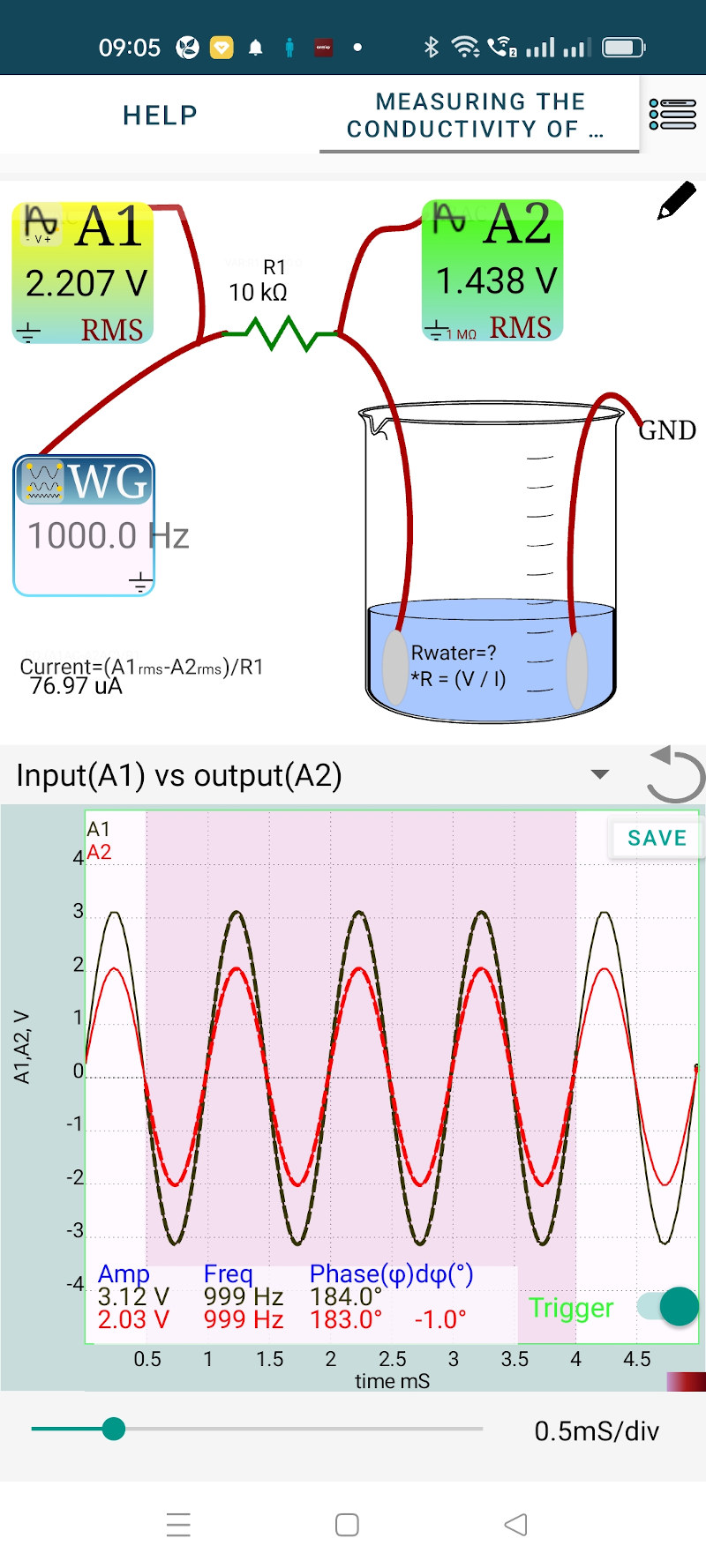# ExpEYES

## Resistivity of Water, using AC

Resistivity of water is an indication of the purity of it, dissolved salts reduce the resistivity. You may try to measure the resistance of some ordinary tap water in a cup using a multimeter. The reading will not stabilize due to the chemical reactions involved. We are measuring the resistance by comparing t with a known resistance, by applying an AC voltage and measuring the ratio of voltages. 1.438/0.000076

• Connect a 10 kOhm resistor (R1) and the water column in series between WG and ground. If the voltage ratio is very high change the value of the resistor. We should use a value comparable to that of the resistance of the water column.
• Tap on the displayed resistor value to enter the actual value used.
• Connect A1 to WG and A2 to the junction between R1 and water.

Slide to select a region to analyze the voltage waveforms. The amplitudes are displayed inside the graph and the RMS voltages on the A1 and A2 icons. The current through R1 is also calculated and displayed. We can use the RMS values for calculation.

• Resistance of water column = Voltage at A2 / Current through it = 1.438/0.000077 = 18682 Ohms.

To find out the specific resistance, you need to use a water column having a known length and cross section.The figure below shows the results after adding a grain of salt.

Resistance = 0.596 / 0.00016 = 3724 Ohms.

The frequency of WG is changed to 100 Hz but it does not have any affect on the resistance.